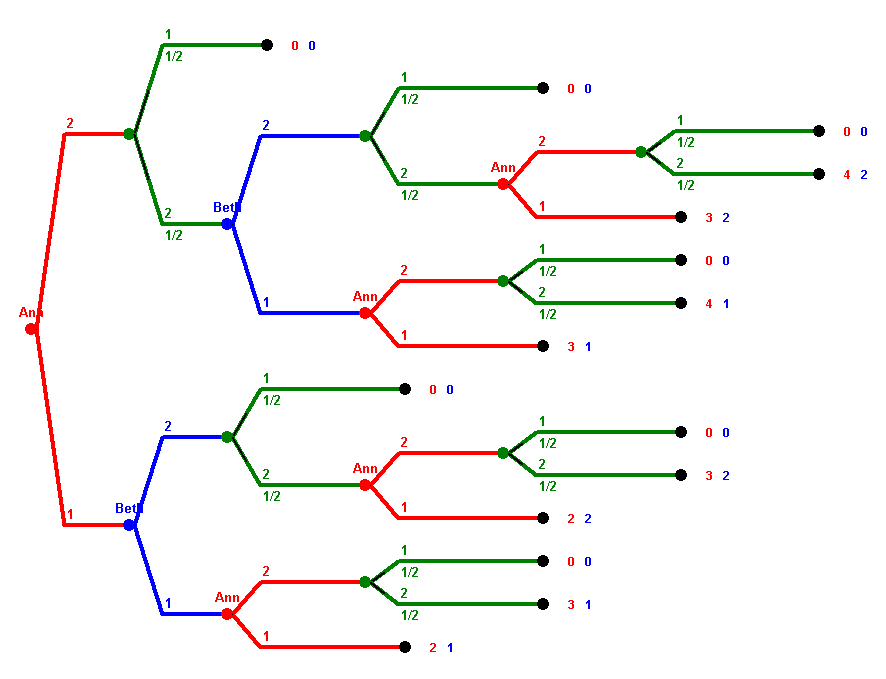# Homework 3

The homework is due on Tuesday, November 25.

1. This question refers to DMA soccer discussed here. Assume each team has six players, and A has three defence, two midfield, and one attack players, and Beth has 2 defence, 2 midfield, and 2 attack players.
• a) What is the probability of a goal for team A in a round?
• b) What is the probability for a goal for team B in a round?
• c) What is the expected value of goals for team A in a 4-round play?
• d) What is the probability for no goal in a round?
• e) What is the probability for a result of 2:1 for team A in a 4-round play?
• f) What is the probability for a result of 1:1 in a 4-round play?
• g) What is the probability for a draw in a 4-round play?
2. Analyse the 3·3 version of Polynomial REC THE SQUARE with randomness game. Explain what will happen if both play optimally. How much will each of the players expect, if a win counts a s +1 and a loss as -1?
Note that you can describe the game using the following 27 states, where each symbol subsumes the cases obtained by rotation and reflection.3. The following sequential game with randomness but perfect information is given in extensive form. There are two players, Ann and Beth. Vertices are labeled by the player whose turn is it. Vertices without such a label are random moves, the probabilities for the two options are 50% and 50% in each case. The payoffs of the end vertices are given, always first Ann's payoff, then Beth's.Analyze the game using backwards induction.
• What first move would Ann make when playing optimally?
• What is the expected payoff for Ann in the game when Ann plays optimally?
4. Consider the following game:

KUHNPOKER: We play with a 8 cards deck, four "1"s and four "2"s. Every player gets one card and looks at it secretly. The start bet is \$2. Ann moves first by either checking or raising.

• If Ann checks, then Beth can check or raise.
• If Beth checks, both cards are revealed and the player with the higher card wins the pot of \$4. splitting it again equally in case of a draw.
• If Beth raises, she increases the bet to \$3. Then Ann has two options, she can either fold or call.
• If Ann folds, Beth gets the pot money of \$5, i.e. wins \$2. Ann's card is not revealed in that case.
• If Ann calls, she also increases her bet to \$3. Then both cards are revealed again, and the player with the higher card gets the money of \$6, i.e. wins \$3. Again, in case of a draw the money is split equally.
• If Ann raises, she increases the bet to \$3. Then Beth has two options, she can either fold or call.
• If Beth folds, Ann gets the pot money of \$5, i.e. wins \$2. Beth's card is not revealed in that case.
• If Beth calls, she also increases her bet to \$6. Then both cards are revealed again, and the player with the higher card gets the money of \$6, i.e. wins \$3. Again, in case of a draw the money is split evenly.

Draw the extensive form of the game. How many pure strategies does Ann have, and how many pure strategies does Beth have?